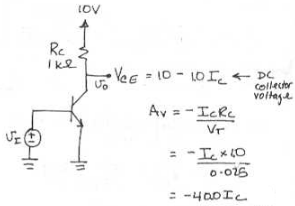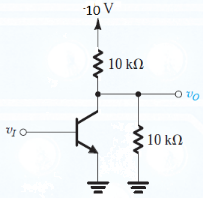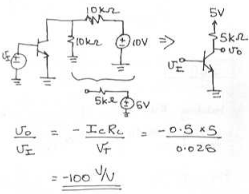Courses

# Test: BJT In Amplifier Design

## 10 Questions MCQ Test Electronic Devices | Test: BJT In Amplifier Design

Description
This mock test of Test: BJT In Amplifier Design for Electrical Engineering (EE) helps you for every Electrical Engineering (EE) entrance exam. This contains 10 Multiple Choice Questions for Electrical Engineering (EE) Test: BJT In Amplifier Design (mcq) to study with solutions a complete question bank. The solved questions answers in this Test: BJT In Amplifier Design quiz give you a good mix of easy questions and tough questions. Electrical Engineering (EE) students definitely take this Test: BJT In Amplifier Design exercise for a better result in the exam. You can find other Test: BJT In Amplifier Design extra questions, long questions & short questions for Electrical Engineering (EE) on EduRev as well by searching above.
QUESTION: 1

Solution:
QUESTION: 2

### The corresponding maximum input signal permitted is

Solution:

If we assume linear operation right to saturation we can use the gain Av to calculate the maximum input signal. Thus for an output swing ∆ Vo = 0.8 we have
∆ Vi = ∆ Vo / Av = -0.7 / -360 = 1.94 mV.

QUESTION: 3

### (Q.3- Q.5) For the amplifier circuit in Fig. 6.33(a) with Vcc = +10 V, Rc = 1 kΩ and the DC collector bias current equal to Ic Q. Find the voltage gain.

Solution:QUESTION: 4

The maximum possible positive output signal swing as determined by the need to keep the transistor in the active region.

Solution:

Assuming the output voltage Vo = 0.3v is the lowest Vce to stay out of saturation.
Vo = 0.3 = 10 – IcRc
= 10 – IcRc + ∆Vo
∆ Vo = -10 + 0.3 + Ic*1.

QUESTION: 5

The maximum possible negative output signal swing as determined by the need to keep the transistor in the active region.

Solution:

Maximum output voltage before the Transistor is cutoff.
Vce + ∆Vo = Vcc
∆Vo = Vcc – Vce
= 10 – 10 + 10 Ic
= 10 Ic.

QUESTION: 6

The transistor in the circuit below is biased at a dc collector current of 0.5 mA. What is the voltage gain?Solution:QUESTION: 7

For a BJt Vt is 5 V, Rc = 1000 ohm and bias current Ic is 12 mA. The value of the voltage gain is __________

Solution:

Voltage gain is (Ic X Rc ) / Vt.

QUESTION: 8

(Q.8–Q.10) (Q.3- Q.5) For the BJT amplifier circuit with Vcc = +10 V, Rc = 1 kΩ and the DC collector bias current equal to 5 mA,

Q. The value of the voltage gain is _______________

Solution:

The voltage is 400 X Ic where Ic is 5 mA.

QUESTION: 9

The maximum possible positive output signal swing as determined by the need to keep the transistor in the active region.

Solution:

The maximum voltage swing is given by -10 + 0.3 + (Ic X Rc). Putting Ic as 5 mA, we get -4.7 mV.

QUESTION: 10

The maximum possible negative output signal swing as determined by the need to keep the transistor in the active region.

Solution:

It is given by -10 + 10 + (Ic X Rc). Putting Ic as 5 mA we get 5V.